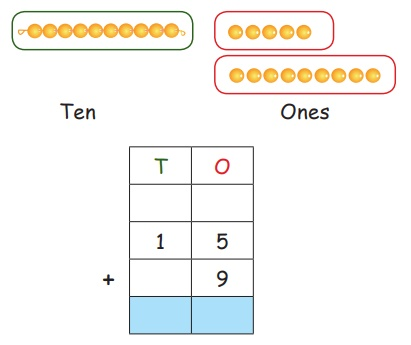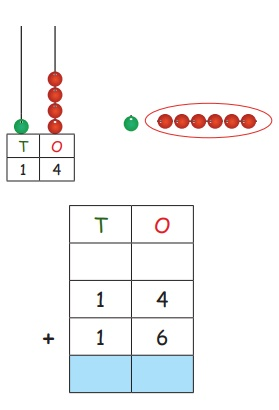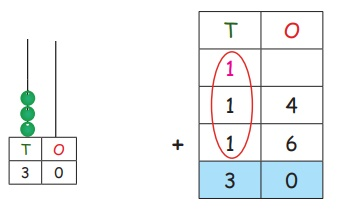Home | | Maths 2nd Std | Addition upto 99 with regrouping

# Addition upto 99 with regrouping

Recalli) 33 + 41 =  74

ii) 52 + 27 = 79

iii) 63 + 24 =  87

iv) 44 + 33 = 77

v) 35 + 23 =  58

vi) 32 + 27 = 59

Travel Through

Agaran, Kuralini, Ezhisai and Agilan played, ‘Blow, Pick and Add’ game using tamarind seeds by forming two teams. Each of the players in the teams were given three chances. Players seperate the tamarind seeds from the heap of seeds by blowing and pick only the seperated seeds by counting them. While collecting the seperated seeds if it touches the other seeds then the player looses his chance and the next player get the chance to play.Number of seeds picked by each of the players is given in the table.The team which collects maximum number of seeds are declared as winner.

Learn

What is the total number of seeds collected by team A in chance 1? Team A consists of two players Agaran and Kuralini. From the table, in chance 1, the seeds collected by them are 15 and 9. So, let us add 15 and 9 using beads, followed by the procedure for addition.

Take 15 seeds and 9 seeds as shown below.Separate the seeds into the groups of tens and ones. Seperate 15 seeds as 1 tens and 5 ones. Take 9 seeds as 9 ones.

We add ones, we get 14 ones.Step 2: Regroup ones

Regrouping ones we get 1 ten + 4 ones. So, write 4 in ones place and carry over 1 ten in tens place.The total number of seeds collected by Team A in chance 1 is 24.

Learn

What is the total number of seeds collected by team B in chance 1? Team B consists of two players Ezhisai and Agilan. They collected 14 and 16 seeds in chance 1. Hence we need to add 14 and 16 using Abacus followed by addition procedure. Put 14 beads in the abacus fi rst and then add 16 beads to it.If we add ones, we get 10 ones.Step 2: Regroup the ones

Regrouping ones we get 1 ten and no ones. So, write 0 in ones place and carry over 1 ten in tens place.Altogether, team B collected 30 seeds in chance 1.

Practice

i) Find the team that wins in chance 2.Team A : 33 + 37 = 70

Team B : 23 + 38 = 61

The team that wins in chance 2 is Team A.

ii) Find the team that wins in chance 3?Team A : 11 + 13 + 10 = 34

Team B : 12 + 12 + 11 = 35

The team that wins in chance 3 is Team B

iii) Find the total number of seeds collected by Agaran and Agilan.Agaran : 15 + 33 + 29 = 77

Agilan : 16 + 38 + 37 = 91

The total number of seeds collected by Agaran is 77.

The total number of seeds collected by Agilan is 91.

Try This

i) Frame the appropriate question for the number fact given in the table (page number 26) of the ‘Blow, Pick and Add’ game.

1. 33 + 37 = 70

Agaran collected 33 seeds.

Kuralini collected 37 seeds.

Find the total number of seeds collected by both of them.

2. 26 + 37 = 63

Ezhisai collected 26 seeds.

Agilan collected 37 seeds.

Find the total numbers of seeds  collected by both of them.Pleasure Time

Fill in the empty boxes given below.Mental maths

1. A vegetable seller packs 72 cauliflowers on the first day and 18 cauliflowers on the second day. Find the total number of cauliflowers packed in two days?

Ans :72 + 18 = 90 cauliflowers

2. Sujitha counts 24 mango trees on one side of the field and Babitha counts 36 mango trees on the other side of the field. What is the total number of mango trees counted?

Ans :24 + 36 = 60 mango trees

3. An ice cream parlour sells 28 ice creams on Monday and 53 ice creams on tuesday. How many ice creams does it sell in two days?

Ans :28 + 53 = 81 ice creams

4. The teacher checks 12 note books on a day. She checks 18 more note books on the next day. What is the total number of note books checked by the teacher on the two days?

Ans :12 + 18 = 30 note books

5. A gardener plucks 28 lemons in the morning and 15 lemons in the afternoon. Find the total number of lemons he plucked.

Ans :28 + 15 = 43 lemon

Think Like A Mathematician

Number puzzlePut the numbers 11, 12, 13, 14, 15, 16 and 17 in the circles so that addition of three numbers in a straight line gives the same total.

Pleasure Time

i) Find the missing digits of the additon facts given below.ii) Raghul gives the sales record sheet to his father to keep a track of the dress materials sold on the first three days of the week.Look at the sales record sheet and find the total number of sarees he sold on the following days by completing the information.Monday 15 + 10 + 20 = 45

Tuesday 25 + 12 + 14 = 51

Wednesday 30 + 13 + 35 = 78

Tags : Numbers | Term 2 Chapter 2 | 2nd Maths , 2nd Maths : Term 2 Unit 2 : Numbers
Study Material, Lecturing Notes, Assignment, Reference, Wiki description explanation, brief detail
2nd Maths : Term 2 Unit 2 : Numbers : Addition upto 99 with regrouping | Numbers | Term 2 Chapter 2 | 2nd Maths